# 为什么OpenGL里的变换矩阵是4x4的？

OpenGL ES 的很多教程里都会有这样一个例子来讲解纹理：将一张图片作为纹理显示在屏幕上。

$\begin{bmatrix} cos\theta&-sin\theta&0&0\\ sin\theta&cos\theta&0&0\\ 0&0&1&0\\ 0&0&0&1 \end{bmatrix}$

## 向量和矩阵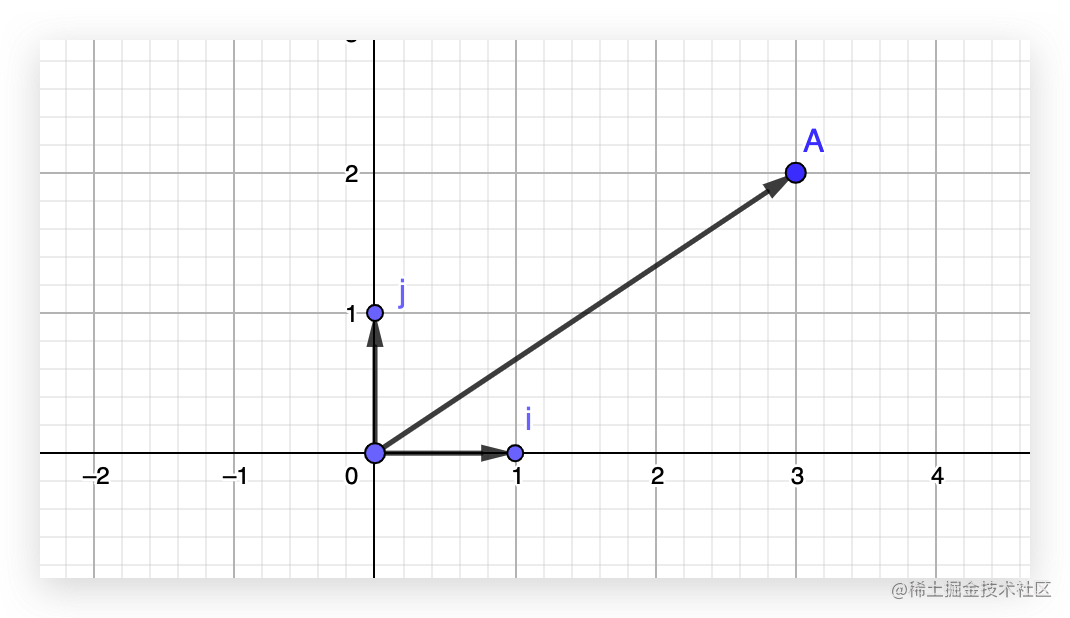$3\vec{i} + 2\vec{j} = \vec{OA}$

i 坐标是 （1， 0），j 坐标是 （0，1），我们把上面这个等式转换成竖列的形式：

$3 \begin{bmatrix} 1\\0 \end{bmatrix} + 2 \begin{bmatrix} 0\\1 \end{bmatrix} = \begin{bmatrix} 3\\2 \end{bmatrix}$

$\begin{bmatrix} 3 \times 1\\ 3 \times 0 \end{bmatrix} + \begin{bmatrix} 2 \times 0\\ 2 \times 1 \end{bmatrix} = \begin{bmatrix} 3 \times 1 + 2 \times 0\\ 3 \times 0 + 2 \times 1 \end{bmatrix} = \begin{bmatrix} 3\\ 2 \end{bmatrix}$

$\begin{bmatrix} 1 & 0\\ 0 & 1 \end{bmatrix} \begin{bmatrix} 3\\ 2 \end{bmatrix} = \begin{bmatrix} 3 \times 1 + 2 \times 0\\ 3 \times 0 + 2 \times 1 \end{bmatrix} = \begin{bmatrix} 3\\2 \end{bmatrix}$

## 推导旋转矩阵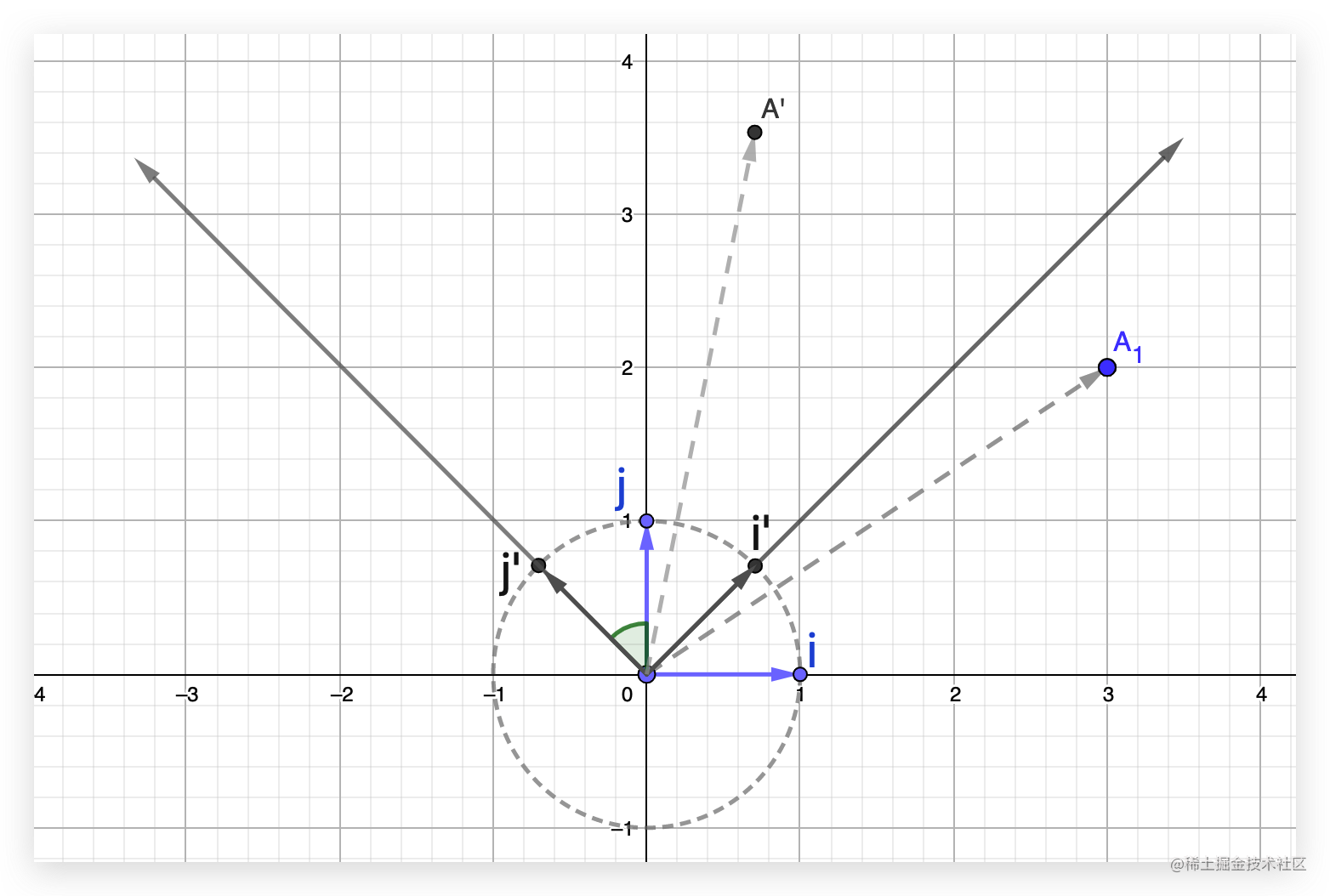$\begin{bmatrix} cos45^o & -sin45^o \\ sin45^o & cos45^o \end{bmatrix} \begin{bmatrix} 3 \\ 2 \end{bmatrix}$

$\begin{bmatrix} cos\theta & -sin\theta \\ sin\theta & cos\theta \end{bmatrix}$

• 变换前后，直线仍然保持是直线的状态

• 变换前后，原点保持固定，不会变化

$\begin{bmatrix} 1 & 0 & 0 \\ 0 & 1 & 0 \\ 0 & 0 & 1 \end{bmatrix} \\$

$\begin{bmatrix} cos\theta&-sin\theta&0\\ sin\theta&cos\theta&0\\ 0&0&1 \end{bmatrix}$

## 推导平移矩阵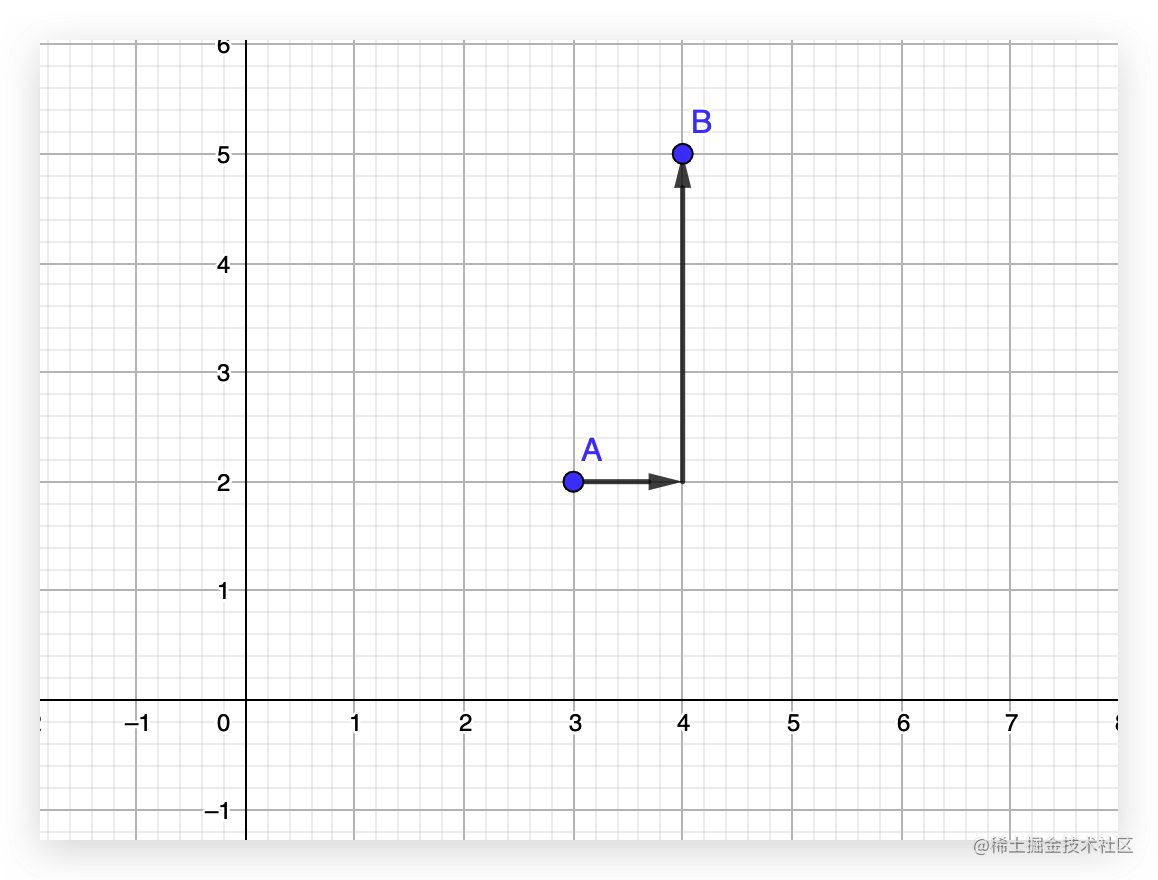$\begin{bmatrix} x + 1 \\ y + 3 \end{bmatrix} = \begin{bmatrix} x \\ y \end{bmatrix} + \begin{bmatrix} 1 \\ 3 \end{bmatrix} = \begin{bmatrix} 3 + 1 \\ 2 + 3 \end{bmatrix} = \begin{bmatrix} 4 \\ 5 \end{bmatrix}$

$\vec{OA} + \vec{OC} = \vec{OB}$
$\begin{bmatrix} 3 \\ 2 \end{bmatrix} + \begin{bmatrix} 1 \\ 3 \end{bmatrix} = \begin{bmatrix} 4 \\ 5 \end{bmatrix}$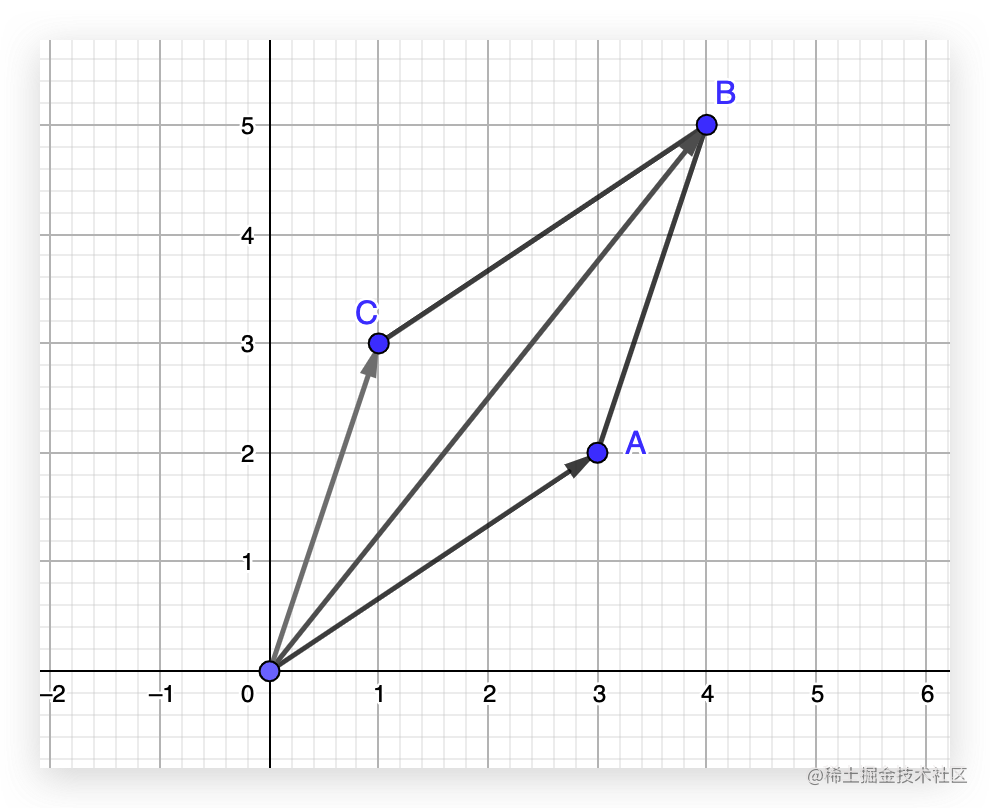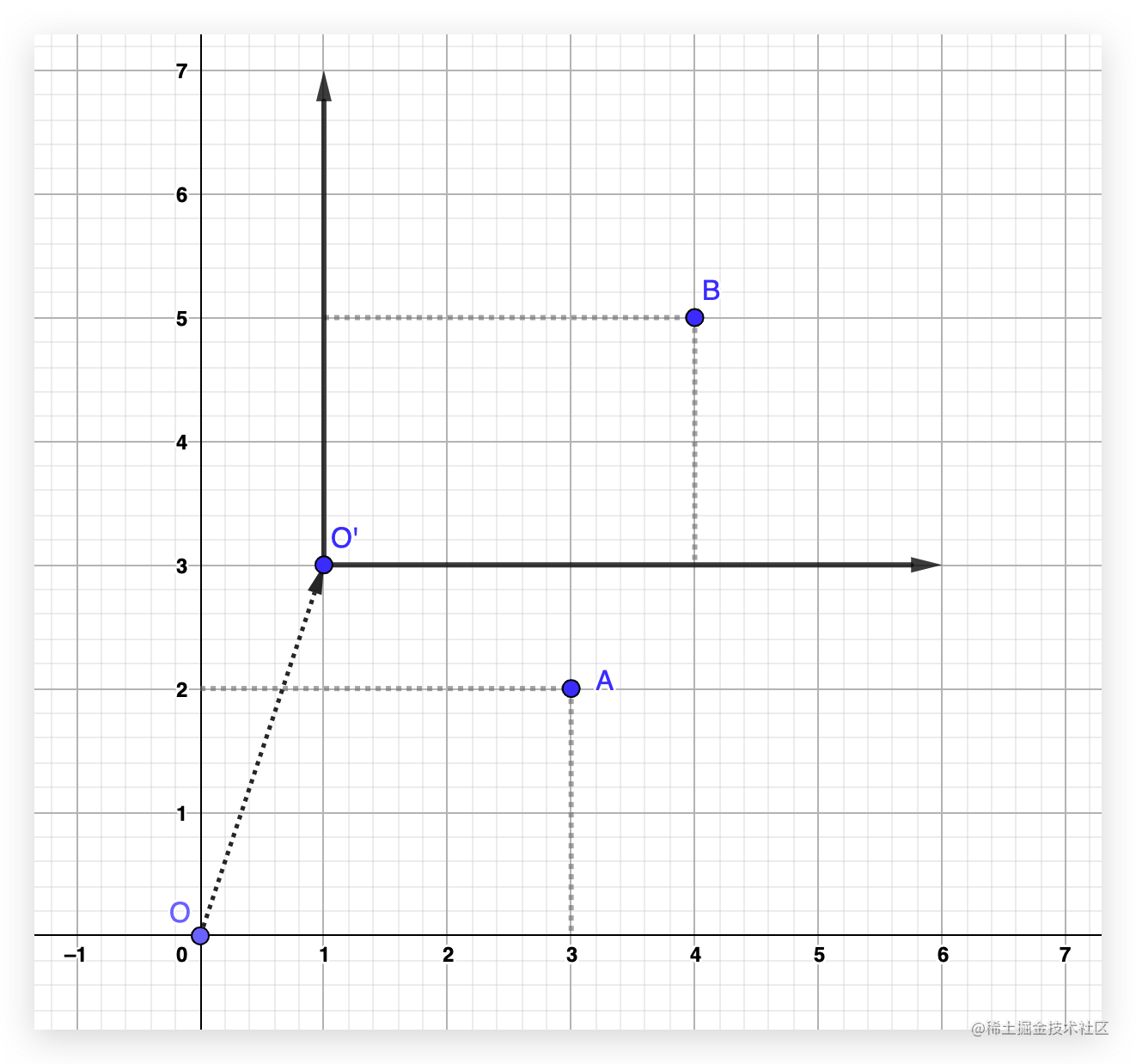$\begin{bmatrix} 矩阵 \end{bmatrix} \begin{bmatrix} 3\\2 \end{bmatrix} = \begin{bmatrix} 4\\5 \end{bmatrix}$

$\begin{bmatrix} 1 & 0 & 1 \\ 0 & 1 & 3 \\ 0 & 0 & 1 \end{bmatrix} \begin{bmatrix} 3 \\ 2 \\ 1 \end{bmatrix} = \begin{bmatrix} 1 \times 3 + 0 \times 2 + 1 \times 1 \\ 0 \times 3 + 1 \times 2 + 3 \times 1 \\ 0 \times 3 + 0 \times 2 + 1 \times 1 \end{bmatrix} = \begin{bmatrix} 4 \\ 5 \\ 1 \end{bmatrix}$

$\begin{bmatrix} 1 & 0 & tx \\ 0 & 1 & ty \\ 0 & 0 & 1 \end{bmatrix}$

tx 和 ty 就对应在 x 轴 和 y 轴上的移动距离。

$\begin{bmatrix} 1 & 0 & 0 & tx \\ 0 & 1 & 0 & ty \\ 0 & 0 & 1 & tz \\ 0 & 0 & 0 & 1 \end{bmatrix}$